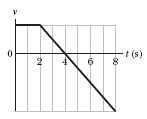# Free Fall Acceleration Problem

## Homework Statement

As a runaway scientific balloon ascends at 19.6 m/s, one of its instrument packages breaks free of a harness and free-falls. The figure below gives the vertical velocity of the package versus time, from before it breaks free to when it reaches the ground.(a) What maximum height above the break-free point does it rise?
(b) How high is the break-free point above the ground?

## The Attempt at a Solution

i know part A is 19.6 m
i just can't seem to get the correct answer for part B

these are the variables that i have and i think i'll need
V0=0 m/s
t=6.0 s
a=-9.8 m/s/s
y-y0=?

i used this kinematic equation
y-y0=vt-1/2at^2
but the answer still isn't correct

kuruman
Homework Helper
Gold Member
The package is at the break-free point at time t = 2 s and it is moving up. Can you figure out by looking at the graph at what later time it is at the same point, moving down?

it's at the same point at t=4 s

kuruman